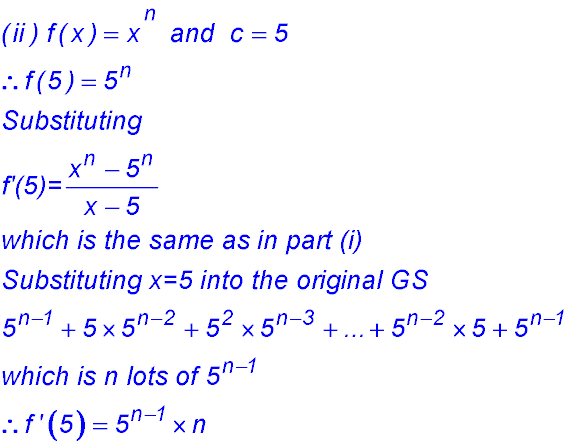Dr. J's Maths.com
Where the techniques of Maths
are explained in simple terms.

Sequences & Series - Geometric - Summation questions.
Test Yourself 1 - Solutions.

 Random S1 = T1 = 9 S2 = T1 + T2 = 25 - so T2 = 16 S3 = 50 = S2 + T3 - so T3 = 25 The first three terms in the series are 9, 16 and 25. Finding the sum. Finding a term. 7.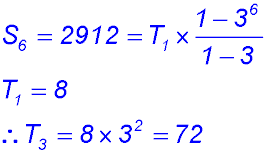8.9.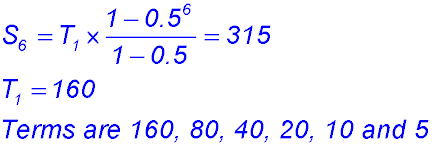Finding the number of terms. 15.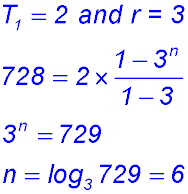16.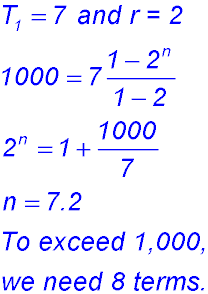Miscellaneous - finding more than one value. 21.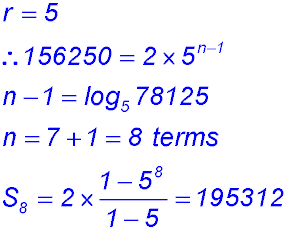22.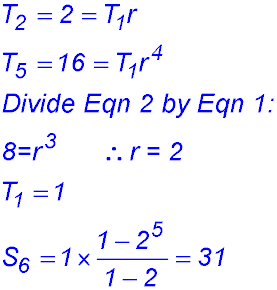Challenging 23.